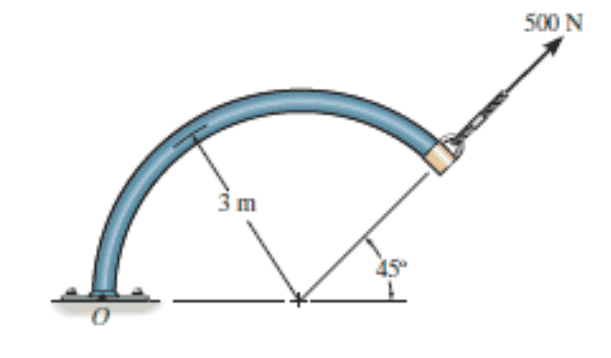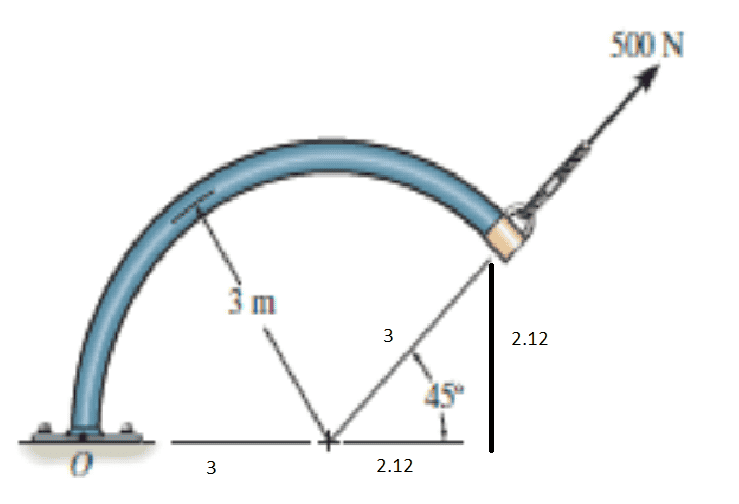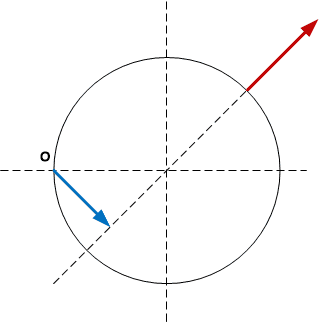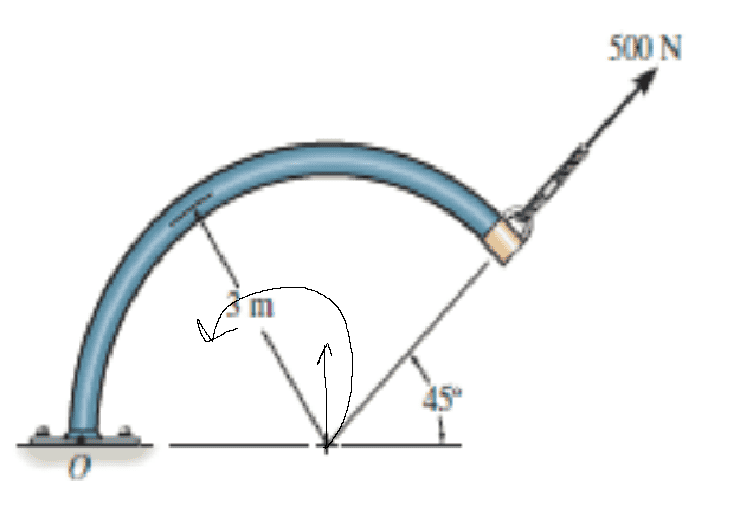# Determine the moment of the force about point O

• sHatDowN
I had a momentary brain cramp. The torque is indeed counter-clockwise. Good catch!In summary, the conversation discusses the components of a 500N force, its horizontal and vertical components (500cos(45) and 500sin(45)), a radius of 3, and the calculation of the moment of the force about point O. The moment is determined by multiplying the vertical component by the horizontal moment arm and subtracting the product of the horizontal component and the horizontal moment arm. The final answer is 1060.65 Nm, with counter-clockwise rotation being the convention.f

#### sHatDowN

Homework Statement
Determine the moment of the force about point O.
Relevant Equations
M = F.dThere are components of 500N:

500cos(45)= 353.55
500sin(45)= 353.55M = (353.55*5.12) - (353.55*2.12) = 1060.65

is that correct?

Homework Statement: Determine the moment of the force about point O.
Relevant Equations: M = F.d

View attachment 324887

There are components of 500N:

500cos(45)= 353.55
500sin(45)= 353.55

View attachment 324888

M = (353.55*5.12) - (353.55*2.12) = 1060.65

is that correct?
It's always good practice to show (or state) your convention with the diagram, and show units in computation. Sig figs are probably too many as well. In this case you chose counter-clockwise as positive moment.

Computationally...the calculation is correct.

Last edited:
•sHatDowN
Yes. It's a bit cumbersome that way. More straightforward:The blue vector is ##{1\over 2}r\sqrt 2##. That times the 500 from ##F## is 1061 Nm

##\ ##

•sHatDowN, erobz and berkeman
It's always good practice to show (or state) your convention with the diagram, and show units in computation. Sig figs are probably too many as well. In this case you chose counter-clockwise as positive moment.

Computationally...the calculation is correct.
Thanks alot.

•berkeman and erobz
M = (353.55*5.12) - (353.55*2.12) = 1060.65
So you are splitting the applied force into its vertical and horizontal components. You computed the torque from the vertical component by multiplying by the horizontal component of the moment arm for the point of application (5.12, 2.12). By inspection, this is a counter-clockwise torque.

You computed the torque from the horizontal component by multiplying against the horizontal moment arm to the same point of application (5.12, 2.12). This time the torque is clockwise, so it will subtract.

That is a viable approach. Straight, by the book, crank and grind.

The approach that I took was different.

The torque from a given force is the same no matter where that force is applied, as long as the revised point of application is somewhere along the "line of action" of the original force.

The drawing makes it clear that the line of action passes through the point (3.00, 0). That simplifies the math. Now the vertical moment arm is zero and we need only consider the vertical force component of 353.55 and the horizontal moment arm of 3.00:$$3.00 * 353.55 = 1060.65$$By inspection, this is a clockwise counter-clockwise torque.

Last edited:
•sHatDowN
The drawing makes it clear that the line of action passes through the point (3.00, 0). That simplifies the math. Now the vertical moment arm is zero and we need only consider the vertical force component of 353.55 and the horizontal moment arm of 3.00:By inspection, this is a clockwise torque.
I think you wrong it's counter-clouckwise becuase in this case when we applied a force horizontal it's counter-clouckwise.•jbriggs444
it's counter-clockwise
I agree.

•sHatDowN
I think you wrong it's counter-clouckwise becuase in this case when we applied a force horizontal it's counter-clouckwise.
You are right, of course.

•sHatDowN and berkeman